# C Program to Find the Length of a String

In this tutorial you will learn about the C Program to Find the Length of a String and its application with practical example.

## C Program to Find the Length of a String.

In this tutorial, we will learn to create a C program that will Find the Length of a String in C programming.

## Prerequisites

Before starting with this tutorial we assume that you are best aware of the following C programming topics:

• Operators in C Programming.
• Basic Input and Output function in C Programming.
• Basic C programming.
• Concepts of for loop.
• Using String functions of c language.

## Find the Length of a String.

As we all know the String is a collection of character data types. In strings, only one variable is declared which can store multiple values. First will take the string from the user. Then will pass that string to a for loop for copying. The C programming language has many pre-defined functions for string manipulation. but in today’s tutorial, we will do it with the help of for loop.

With the help of this program, we can Find the Length of a String.

## Output:-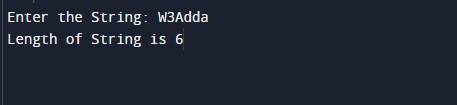In the above program, we have first initialized the required variable.

• string1 = it will hold the string value.
• string2 = it will hold the string value.
• i = it will hold the integer value for the loop.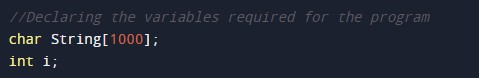Taking input string from the user.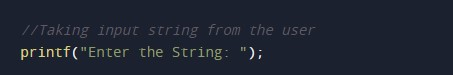Reading the input string from the user/Storing the input from the user.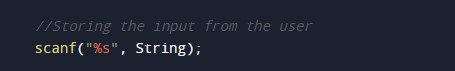Find the length using for loop of a given string.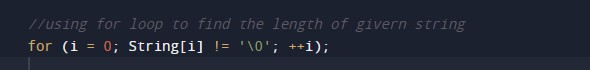Printing the length of the string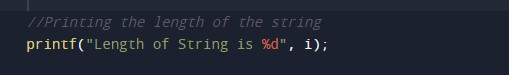In this tutorial we have learn about the C Program to Find the Length of a String and its application with practical example. I hope you will like this tutorial.